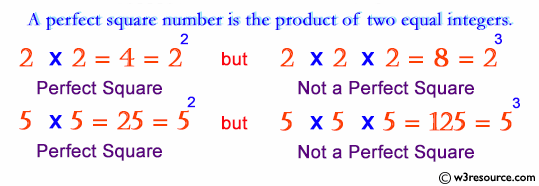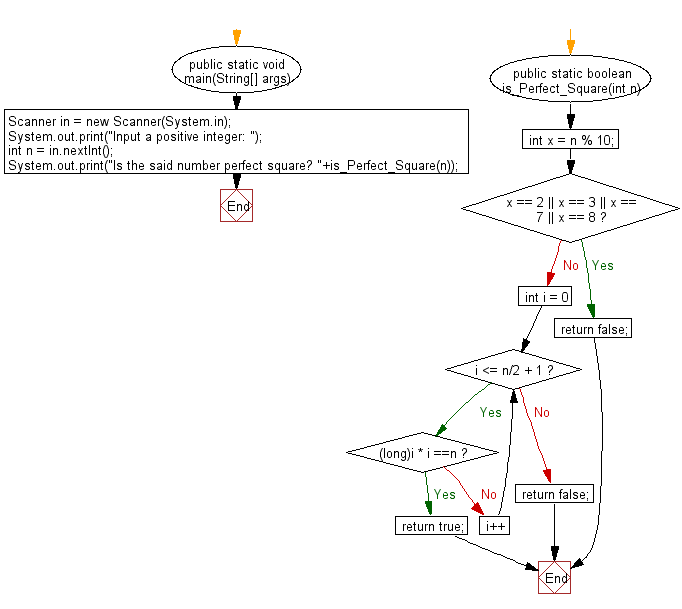# Java Exercises: Test if a given number is a perfect square or not

## Java Basic: Exercise-197 with Solution

Write a Java program to test if a given number (positive integer ) is a perfect square or not.

Input number: 3 Output: 1 2 3 8 9 4 7 6 5

Pictorial Presentation:Sample Solution:

Java Code:

``````import java.util.*;
public class Solution {
public static void main(String[] args) {
Scanner in = new Scanner(System.in);
System.out.print("Input a positive integer: ");
int n = in .nextInt();
System.out.print("Is the said number perfect square? " + is_Perfect_Square(n));
}

public static boolean is_Perfect_Square(int n) {
int x = n % 10;
if (x == 2 || x == 3 || x == 7 || x == 8) {
return false;
}
for (int i = 0; i <= n / 2 + 1; i++) {
if ((long) i * i == n) {
return true;
}
}
return false;
}
}
```
```

Sample Output:

```Input a positive integer:  6
Is the said number perfect square? false
```

Flowchart:Java Code Editor:

Company:

What is the difficulty level of this exercise?

﻿

## Java: Tips of the Day

Parsing dates:

```import java.io.*;
import java.util.*;
import java.text.*;

String s = "2001/09/23 14:39";

SimpleDateFormat formatter = new SimpleDateFormat ("yyyy/MM/dd H:mm");
Date d = formatter.parse(s, new ParsePosition(0));
```